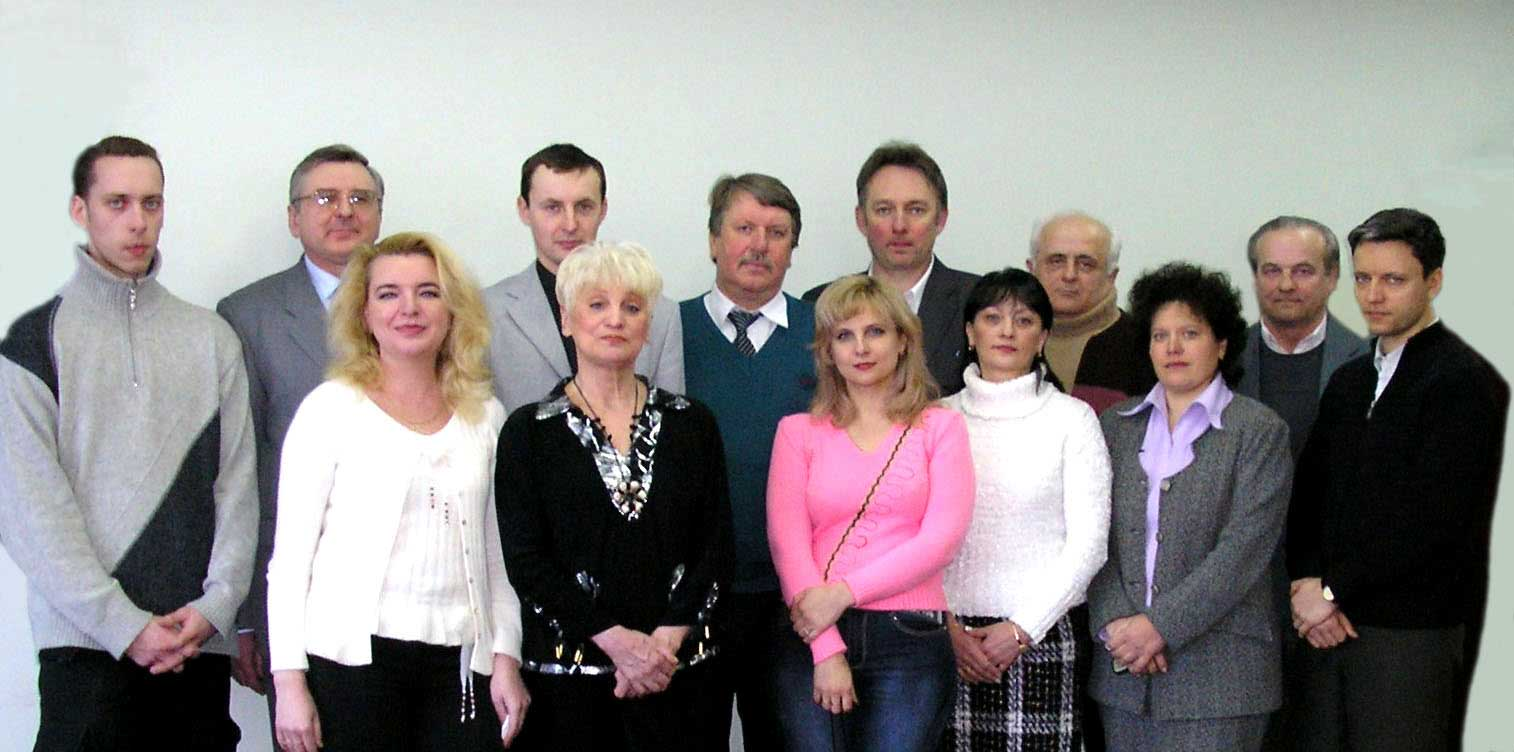### Department AS

Head — Irina V. Rozora - Assoc. Prof., Doctor of Physical and Mathematical SciencesThe department specializes in the following fields: stochastic analysis of systems, theory of statistical decisions, data analysis, the modeling of random processes and fields, optimal control, mass service systems and networks, financial and actuarial mathematics.
The department trains bachelors under the educational program "System Analysis" and masters under the educational program "Systems and methods of decision-making".
Probability theory, mathematical statistics, data analysis, actuarial mathematics, econometrics, methods and models of social information processing, mathematics of finance, risk analysis and optimization, mass service networks, Bayesian networks, mathematical models of insurance and pension schemes.

### Department of Applied Statistics

8

#### Teachers

8 full-time employees including 1 professor, 5 associate professors. 1 assistant. Among them are 2 doctors of sciences and 6 PhDs

25

#### Courses

The employees of the department read 25 regulatory courses

45

#### Years

The Department of Applied Statistics was established on June 7, 1978

### Staff:

Full name: Office Research interests
Prof. Pavlo S. Knopov 709 statistical decision theory, methods of stochastic optimization, theory of optimal control of stochastic systems
Prof. Mykhaylo M. Savchuk 709 probabilistic combinatorics, mathematical methods of information security, cryptography and cryptoanalysis, applied statistics
Assoc. Prof. Alexander S. Slabospitsky 706 б estimation theory, data analysis, applied statistics, regression analysis, analysis of variance, correlation analysis, system identification theory, parameter/state estimation of technical objects, recurrent algorithms of system identification, set membership estimation, real-time data processing, system modelling, asymptotic analysis of estimation and identification algorithms, software for analysis and processing of data, computer networks, Internet-technologies
Assoc. Prof. Michael M. Sharapov 706 б limiting theorems for estimates of parameters of random processes and fields with long memory, modeling of random processes and fields with long memory, random walks on graphs, combinatorial solutions of linear programming task, computer realizations of statistical algorithms
Assoc. Prof. Irina V. Rozora 709 property investigation of stochastic processes and fields, modelling of random processes with given accuracy and reliability in various Banach spaces, actuarial mathematics
Assist. Prof. Igor A. Makushenko 709 investigation of stochastic processes of queueing theory, asymptotic analyses of compound stochastic systems, statistical prediction methods
Assoc. Prof. Anna V. Livinska 709 random processes, queueing models, asymptotic analysis of stochastic systems
Prof. Natalia V. Semenova 709 development and research models and methods of discrete optimization, as well as problems with ambiguous data set, stability analysis of vector discrete optimization problems.
Prof. Alexander A. Voina 709 theories of random processes, research in the region statistics of processes with discrete component, development of construction methods optimal strategies management, application mathematical methods cybernetics in economic sciences
Assoc. Prof. Vadym D. Ponomarov 709 random processes, optimal control of stochastic systems, modern programming technologies, evaluation theory
Assoc. Prof. Svitlana A. Ralchenko 709 Probability theory, stochastic differential equations, statistics of random processes, financial mathematics
Assoc. Prof. Olga I. Vasylyk 709 Theory of φ-sub-Gaussian random processes and their application, modeling of random processes, statistics of random processes, actuarial mathematics, mathematical models of risk processes.
Assist. Prof. Viktor V. Semenov 709 development and research of models and methods of discrete optimization and solvability of vector discrete optimization problems.